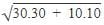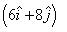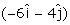NEET  >  Test: Relative Velocity in Two Dimensions

# Test: Relative Velocity in Two Dimensions

Test Description

## 10 Questions MCQ Test Physics Class 11 | Test: Relative Velocity in Two Dimensions

Test: Relative Velocity in Two Dimensions for NEET 2022 is part of Physics Class 11 preparation. The Test: Relative Velocity in Two Dimensions questions and answers have been prepared according to the NEET exam syllabus.The Test: Relative Velocity in Two Dimensions MCQs are made for NEET 2022 Exam. Find important definitions, questions, notes, meanings, examples, exercises, MCQs and online tests for Test: Relative Velocity in Two Dimensions below.
Solutions of Test: Relative Velocity in Two Dimensions questions in English are available as part of our Physics Class 11 for NEET & Test: Relative Velocity in Two Dimensions solutions in Hindi for Physics Class 11 course. Download more important topics, notes, lectures and mock test series for NEET Exam by signing up for free. Attempt Test: Relative Velocity in Two Dimensions | 10 questions in 10 minutes | Mock test for NEET preparation | Free important questions MCQ to study Physics Class 11 for NEET Exam | Download free PDF with solutions
 1 Crore+ students have signed up on EduRev. Have you?
Test: Relative Velocity in Two Dimensions - Question 1

### A boat sails across a river with a velocity of 10 km/hr. If resultant boat velocity is 14 km/hr, then what is the velocity of river water?

Detailed Solution for Test: Relative Velocity in Two Dimensions - Question 1

As the boat moves at 10 kph across the river it is being swept downstream by the river current at a 90 degree angle. resulting i a motion of 14 kph. the downstream velocity of the river can be calculated by the Pythagoras theorem:

10^2+x^=14^2

100+x^2=196

x^2=96

x=9.798 approximately

Test: Relative Velocity in Two Dimensions - Question 2

### The angular speed of the earth about its own axis is:

Detailed Solution for Test: Relative Velocity in Two Dimensions - Question 2

Hour hand i.e- 60x60=3600sec
Time period=2π/angular speed.
T=2π/ ω
ω=2π/60x60=2π/3600
to complete one rotation, time taken=12 hours.
For 12 hours=12x60x60 sec
=43200 sec
Angular speed of hour hand=2π/T
(i.e., a complete round) =2π/43200

Test: Relative Velocity in Two Dimensions - Question 3

### Rain is falling vertically with the speed of 30 m/s. A man rides a bicycle with the speed of 10 m/s in east to west direction. What is the direction in which he should hold the umbrella?

Detailed Solution for Test: Relative Velocity in Two Dimensions - Question 3

When the man rides, he should put an umbrella in the opposite direction to which the drops are falling with respect to him.
Hence wrt man, the speed of rain drop isat an angle of tan-1
Hence he would put umbrella to the direction of tan-1

Test: Relative Velocity in Two Dimensions - Question 4

The angular speed of a wheel of radius 0.50 m moving with the speed of 20 m/s is:

Detailed Solution for Test: Relative Velocity in Two Dimensions - Question 4

Radius r = 0.5 m
Speed, v = 20 m/s
We know,
v = r ω
where,
ω = angular velocity
20 = 0.5 x ω
ω = 20/0.5 = 40 rad/sec

Test: Relative Velocity in Two Dimensions - Question 5

In 5 seconds a body covers a distance of 50 m and in 10 seconds it covers 80 m with uniform acceleration. What is the distance traveled in 15 seconds?

Detailed Solution for Test: Relative Velocity in Two Dimensions - Question 5

Correct Answer :- d

Explanation : Let the initial velocity of the body be u m/s; and the constant acceleration be a m/s². Then displacement (distance covered) of the body after t second is given by

s = u t + ½ a t²       —(1)

The body covers 50 m in 5s and 80 m in 10s.

50 = u×5 + ½ a 5²; => 50 = 5u + 25/2a

10u + 25a = 100     —(2)

80 = u×10 + ½ a 10²; => 80 = 10u + 50a; this gives,

u + 5a = 8  —(3)

Solving (2) and (3) for u and a we get

u = 12 m/s and a = -4/5 m/s²  — (4)

To obtain the distance covered in the next 15s we need to find the velocity at the beginning of this interval using,

v = u + a t => v = 12 m/s + (-4/5) m/s² × 15s = 0m/s

s = u t + ½ a t²

Substituting various values we get

s = 12×15 +½ ×(-4/5)×15² = 180 - 90

= 90 m

Test: Relative Velocity in Two Dimensions - Question 6

The angular speed of a flywheel making 210 revolutions per minute is:

Detailed Solution for Test: Relative Velocity in Two Dimensions - Question 6

As the flywheel makes 210 revolution in a minute, and during each revolution the angular displacement of the flywheel  is 2π thus total angular distance covered is
210 × 22/7 × 2 = 1320 rad
Therefore angular speed=1320 rad/60 s = 22 rad/s

Test: Relative Velocity in Two Dimensions - Question 7

A boat is moving with a velocitywith respect to the ground. The water in the river is moving with a velocitywith respect to the ground. What is the relative velocity of boat with respect to river?

Detailed Solution for Test: Relative Velocity in Two Dimensions - Question 7

Velocity of boat wrt river = velocity of boat wrt ground - velocity of river wrt ground
I.e. v = (6i + 8j) - (-6i - 4j)
= 12i + 12j

Test: Relative Velocity in Two Dimensions - Question 8

The angular speed of the second hand of a watch is :

Detailed Solution for Test: Relative Velocity in Two Dimensions - Question 8

The second hand goes through 2π radians in 1 min, or 2π radian/60 seconds, so ω = π/30 rad.s-1 = 0.03 rad.s-1

Test: Relative Velocity in Two Dimensions - Question 9

A monkey is running on the ground, next to a train, in the direction of the train. The speed of the monkey is 5km/h and the speed of the train is 100km/h. What is the velocity of the monkey with respect to the train?

Detailed Solution for Test: Relative Velocity in Two Dimensions - Question 9

Assuming the direction of velocity of train to be positive, wrt train the speed of monkey will be
-100 + 5 = -95 kmph
I.. 95 kmph in direction opposite to the train.

Test: Relative Velocity in Two Dimensions - Question 10

The angular speed of a wheel of radius 0.50 m moving with the speed of 20 m/s is:

Detailed Solution for Test: Relative Velocity in Two Dimensions - Question 10

Radius r = 0.5 m

Speed, v = 20 m/s

We know,

v = r ω

where,

ω = angular velocity

20 = 0.5 x ω

ω = 20/0.5 = 40 rad/sec

## Physics Class 11

126 videos|449 docs|210 tests
 Use Code STAYHOME200 and get INR 200 additional OFF Use Coupon Code
Information about Test: Relative Velocity in Two Dimensions Page
In this test you can find the Exam questions for Test: Relative Velocity in Two Dimensions solved & explained in the simplest way possible. Besides giving Questions and answers for Test: Relative Velocity in Two Dimensions, EduRev gives you an ample number of Online tests for practice

## Physics Class 11

126 videos|449 docs|210 tests

### How to Prepare for NEET

Read our guide to prepare for NEET which is created by Toppers & the best Teachers